# FinishedAll about Hidden Power on PRO

#### Kaminokage

##### Veteran Trainer
SUMMARY

• I. Introduction
• II. What is Hidden Power and why should I use it?
• III. What Pokemon can learn Hidden Power and where can I find it?
• IV. How works Hidden Power Type?
• V. Percentage distribution of different variations of Hidden Power
• VI. The Odd/Even Method
• VII. Calculator Hidden Power on Excel
• VIII. ReferencesI. Introduction

We often see in chat a lot of people ask « what is HP ? » or want to sell, for example, a PKM HP Fire when it’s not.
So, this guide has been created for all people: Newbie who want to know what is Hidden Power and regular player who want to chase a PKM with a specific Type for Hidden Power.

It’s the player diabo123 with his guide who gave me the idea to create this guide. I will try to explain what is Hidden Power, why we should use it, and how to recognize the HP Type of a PKM.

In this guide, I will use some abbreviation:
• HP = Hidden Power
• hp = health points
• PKM = PokemonII. What is Hidden Power and why should I use it?

The ingame description of Hidden Power is :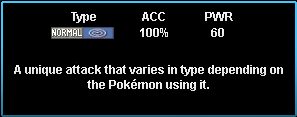Hidden Power appears as a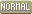Type move but the actual Type of Hidden power varies with the IVs of the PKM.
So, Hidden Power Type can be: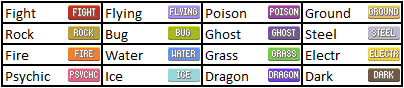You notice that Hidden Power can’t beType or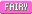Type.

Hidden Power hits in category Special with a Base Power of 60.

So, why should I use Hidden Power?

Hidden Power is a very good move to surprise your opponent, indeed, you will have the choice between 16 Type for HP, so your opponent will never know what did you choose. It’s an excellent move for PKM with a poor movepool (Lilligant, Manectric). It can be used for PKM with the Ability Technician, Hidden Power will hit at 60*1,5 = 90 for any Type (Roserade, M. Mime, Persian). Or, to allow a better cover of types: Electric PKM with HP Ice/Grass/Water to hits Ground PKM.
Hidden Power is often used in PVP, the perfect example is Magnezone with the Ability Magnet Pull: you will trap Steel PKM with Magnet Pull and use HP Fire to hit Steel PKM.III. What Pokemon can learn Hidden Power and where can I find it?

You can find on Bulbapedia what PKM can learn Hidden Power by Leveling up: https://bulbapedia.bulbagarden.net/wiki/Hidden_Power_(move)

By TM, all Pokemon can learn Hidden Power.
(except: Wobbuffet, Wynaut, Kricketot, Tynamo, Spewpa and Pyuhumuku according to Bulbapedia)

The TM 60 can be find in Slateport Outdoor Market for \$4 000: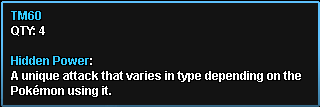IV. How works Hidden Power Type?

I will start the mathematics part, it’s really easy, so don’t stop read now :Sleeping:

To determinate the HP Type, we use the formula: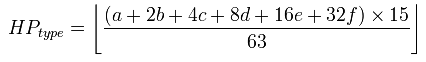With: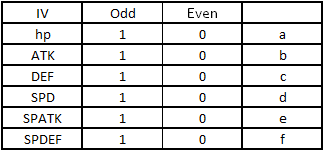It’s a binary system, if the IV hp is equal to 29, it’s an odd number so a = 1, if the IV hp is equal to 28, it’s an even number a = 0. And we do the same with the rest.
In the end, we truncate the result of this formula and we find a number from 0 to 15. And this value give the HP Type: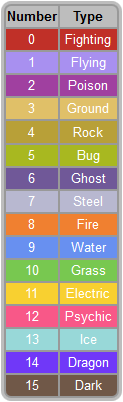I will take an example to understand in practice:

I just catch this Magnemite, and I want to know what his HP Type is.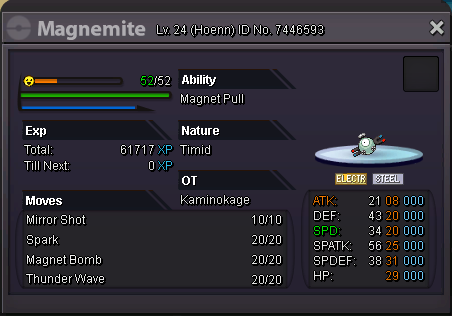In first, find the value of a, b, c, d, e and f: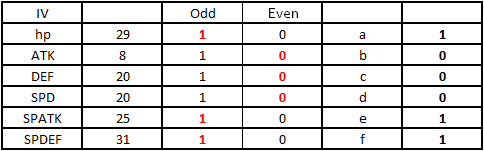or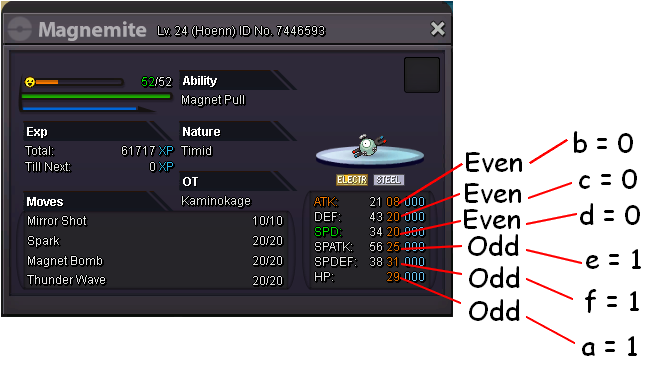Now, I have the value of a, b, c, d, e and f, I just have to fill the formula :HP Type = floor [ ( a + 2 b + 4 c + 8 d + 16 e + 32 f ) * 15/63 ]
HP Type = floor [ ( 1 + 2 * 0 + 4 * 0 + 8 * 0 + 16 * 1 + 32 * 1 ) * 15/63 ]
HP Type = floor [11,67]
HP Type = 11
So, I look what is number 11, and it’s Electric. This Magnemite is HP Electric.

In practice, to know what is your HP Type, you can use a calculator find on google : https://www.psypokes.com/dex/hp.php
You will see a Base Power for Hidden Power given by the calculator, in previous generation, the Type was determinate by the IVs with a Mod 4 in the formula. In PRO, it fixes to 60, so you don’t need it.
Or use the Calculator i made : http://fsogame.free.fr/tools/calchptype.phpV. Percentage distribution of different variations of Hidden Power

Now, you know what Hidden Power is and how recognize the HP Type, you want to chase a PKM with a Specific Type. But stop! You have to know some probabilities before spend some hours of farm.
I can link you Bulbapedia with his Percentage distribution, but this one is wrong for PRO. Indeed, for official game, the IVs are from 0 to 31, in PRO, the IVs are from 1 to 31. It means the distribution odd-even is not equitable. So, the percentage distribution will not be the same.

How can I calculate that?
It’s easy, there are 2^6 = 64 different combination of HP Type, for each, I just have to calculate the probability and affect it to the Type: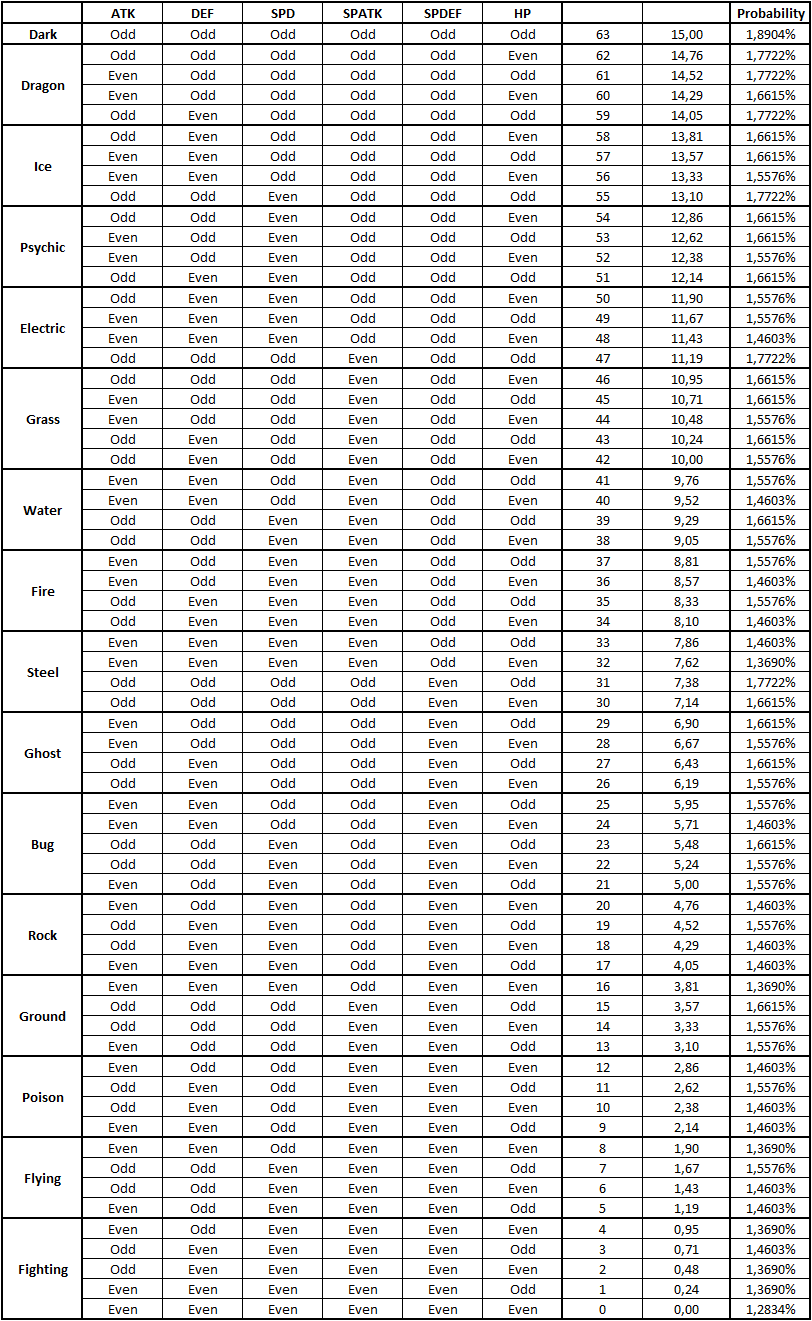To resume the percentage distribution of different variations of Hidden Power :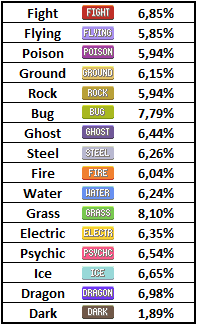This distribution means that if you want to chase Magnemite HP Fire, you will catch only 6,04% of HP Fire when you will look in PC.
Or, before you chase Bulbasaur H.A. HP Fire, you had to know that H.A. is only 5% and HP Fire is 6,04%, so only 0,3% of Bulbasaur will be H.A. HP Fire :devil:VI. The Odd/Even Method

This part of the guide has one goal : make you gain time when you check your PKM.
For each Type, I will explain a method to recognize it. No need to learn all in practice, you will learn only the method for the HP Type you want. But, this methods are not perfect, so i make a note for each:

• Method note: Good => This method can be used easy.
• Method note: Moderate => This method can be used but it's harder.
• Method note: Bad => This method need to use a calculator after.

By the way, if you find a better method than the one i post, you can tell me. I am only human after all, i can need so help :)

Before anything, I will take an example to explain how it works.

Imagine I just catch 450 Magnemite (I did it in practice so it happens :p), I want to see what Magnemite are HP Fire. But I don’t want to enter all IVs in the calculator, what should I do? I will learn only this:
ATK + DEF = odd
SPD = even
SPATK = even
SPDEF = odd
hp = any

How to use it?

I will look 2 Magnemite :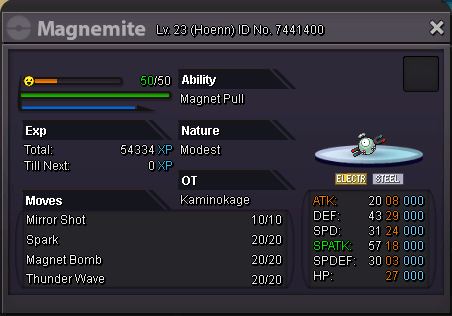ATK + DEF= 8 + 29 = 37 = odd => Ok
SPD = 24 = even => Ok
SPATK = 18 = even => Ok
SPDEF = 3 = odd => Ok
hp = 27 => Ok

So this Magnemite is HP Fire, it takes 3 seconds with only head.

And my other Magnemite :ATK + DEF = 8 + 20 = 28 = not odd => Not HP Fire.
It takes less than 1 second.

So with the Odd/Even method, in 3 seconds, you will see for a PKM if he is HP Fire or not :y:

It's nice but it's for a good method, why should i use a bad method?

Even if a bad method is not perfect because you will have to use a Calculator, this method will reduce the number of PKM you will check. Imagine i want a PKM HP Dragon and i just catch 200 of this PKM, i don't want to check all. So, with the bad method, i will saw 8 combinations on the 64 combinations that can be HP Dragon. I will know that the 56 others combinations can't be HP Dragon so i can release this others combinations. 56/64 means 87,5% of PKM, so i only need to check 12,5% ! So, for 200, i need to check only 25 PKM in average, not 200.

Hidden Power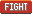: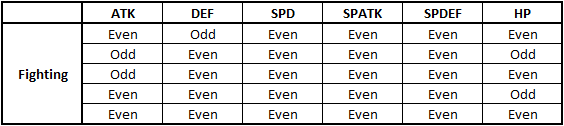To learn :
• DEF / SPD / SPATK / SPDEF = all even
• ATK = any
• hp = any

And another case :
• ATK / SPD / SPATK / SPDEF / hp = all even
• DEF = odd

Method note: Moderate

Hidden Power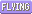: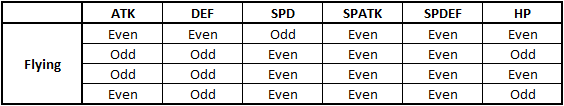To learn :
• DEF + SPD = odd
• SPATK = even
• SPDEF = even

After, you have to use a Calculator : http://fsogame.free.fr/tools/calchptype.php

Hidden Power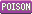: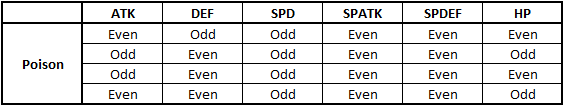To learn :
• SPD = odd
• SPATK = even
• SPDEF = even
• ATK / DEF / hp = One have to be Odd, the two others have to be Even

And another case :
• ATK = odd
• DEF = even
• SPD = odd
• SPATK = even
• SPDEF = even
• hp = odd

Method note: Moderate

Hidden Power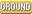: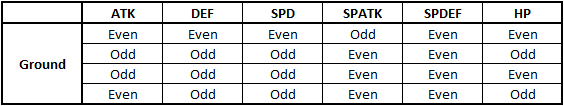To learn :
• SPD + SPATK = odd
• SPDEF = even

After, you have to use a Calculator : http://fsogame.free.fr/tools/calchptype.php

Hidden Power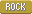: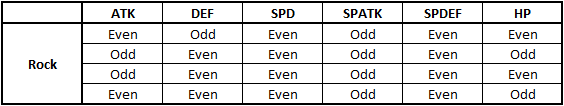To learn :
• SPD = even
• SPATK = odd
• SPDEF = even
• ATK / DEF / hp = One have to be Odd, the two others have to be Even

And another case :
• ATK = odd
• DEF = even
• SPD = even
• SPATK = odd
• SPDEF = even
• hp = odd

Method note: Moderate

Hidden Power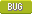: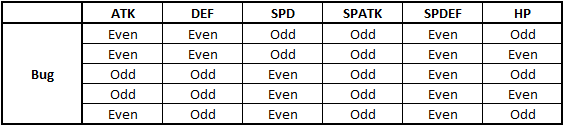To learn :
• ATK + DEF = even
• DEF + SPD = odd
• SPATK = odd
• SPDEF = even
• hp = any

And another case :
• ATK = even
• DEF = odd
• SPD = even
• SPATK = odd
• SPDEF = even
• hp = odd

Method note: Moderate

Hidden Power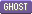: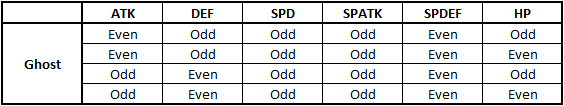To learn :
• ATK + DEF = odd
• SPD = odd
• SPATK = odd
• SPDEF = even
• hp = any

Method note: Good

Hidden Power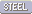: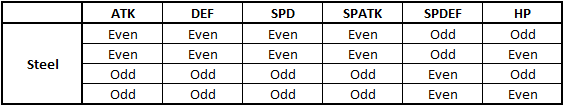To learn :
• ATK / DEF / SPD / SPATK = all even
• SPDEF = odd
• hp = any

And To learn :
• ATK / DEF / SPD / SPATK = all odd
• SPDEF = even
• hp = any

Method note: Good

Hidden Power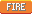: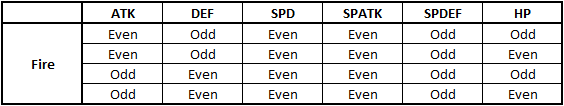To learn :
• ATK + DEF = odd
• SPD = even
• SPATK = even
• SPDEF = odd
• hp = any

Method note: Good

Hidden Power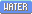: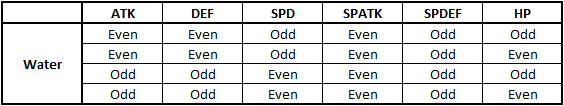To learn :
• ATK + SPD = odd
• DEF + SPD = odd
• SPATK = even
• SPDEF = odd
• hp = any

Method note: Good

Hidden Power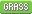: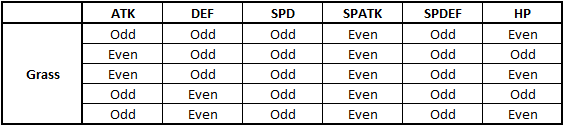To learn :
• SPD = odd
• SPATK = even
• SPDEF = odd
• ATK + DEF = odd
• hp = any

And another case :
• SPD = odd
• SPATK = even
• SPDEF = odd
• ATK / DEF = all odd
• hp = even

Method note: Moderate

Hidden Power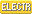: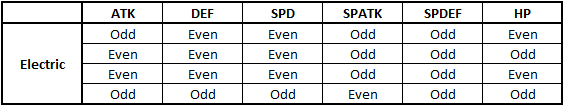To learn :
• SPDEF = odd
• DEF + SPATK = odd
• SPD + SPATK = odd

After, you have to use a Calculator : http://fsogame.free.fr/tools/calchptype.php

Hidden Power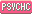: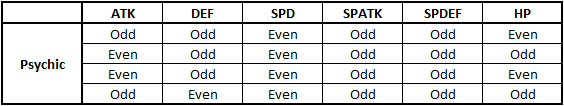To learn :
• SPD = even
• SPATK = odd
• SPDEF = odd
• ATK = odd
• DEF + hp = odd

And To learn :
• SPD = even
• SPATK = odd
• SPDEF = odd
• ATK = even
• DEF = odd
• Hp = any

Method note: Moderate

Hidden Power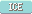:To learn :
• DEF + SPD = odd
• SPATK = odd
• SPDEF = odd

After, you have to use a Calculator : http://fsogame.free.fr/tools/calchptype.php

Hidden Power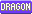: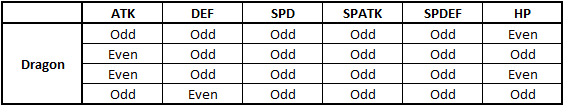To learn :
• SPD = odd
• SPATK = odd
• SPDEF = odd

After, you have to use a Calculator : http://fsogame.free.fr/tools/calchptype.php

Hidden Power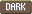: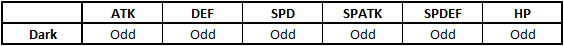To learn :
• ATK / DEF / SPD / SPATK / SPDEF / hp = all odd

Method note: GoodVII. Calculator Hidden Power on Excel

I share the Excel i use for Hidden Power. When i chase a PKM with different HP Type possible, i prefer use Excel than an Internet Calculator.
https://www.dropbox.com/s/f9bbnaqobwa89kc/CALCULATOR HIDDEN POWER.xlsx?dl=0
In the right top, you can click on download. If something is wrong with the Excel or the uploader, tell me.

To use it, just fill cells with the IVs, my excel gives you the TOTAL 6 IVs and the Hidden Power Type: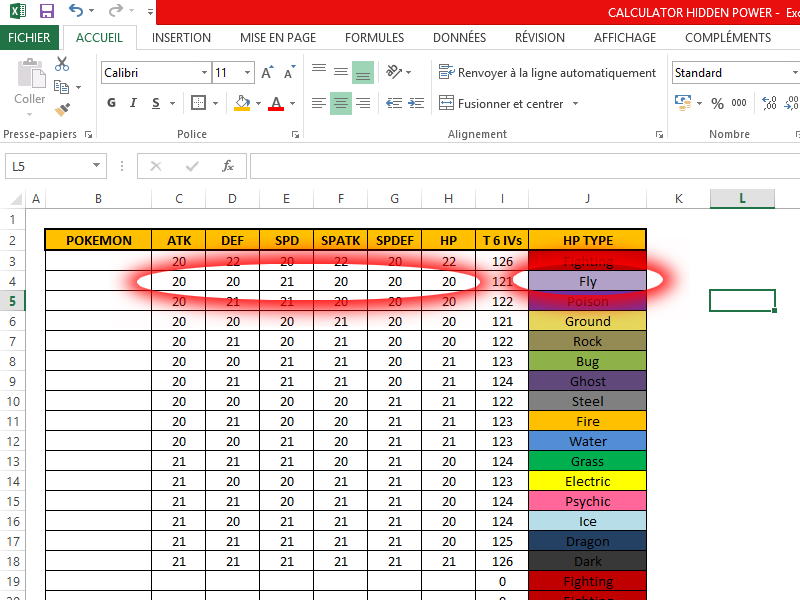VIII. References

Bulbapedia Hidden Power (Move) : https://bulbapedia.bulbagarden.net/wiki/Hidden_Power_(move)
Bulbapedia Hidden Power (Calculation) : https://bulbapedia.bulbagarden.net/wiki/Hidden_Power_(move)/Calculation
HP Type Calculator : https://www.psypokes.com/dex/hp.php
HP Type Calculator by Kaminokage : http://fsogame.free.fr/tools/calchptype.phpThanks you for reading, if you see some errors or want to add something to this post, don't hesitate to contact me :)

And sorry for my english, it's not my native language :(

Last edited:
•#### chameleon

PRO Member
Donator
Re: All about Hidden Power in PRO

<t>Great work on the guide, it's very helpful. Thanks a lot!</t>

Donator
well done :)

Nice work :y:

#### m0atz

##### Suspended
Suspended
Good job bro :Angel:

#### Kaminokage

##### Veteran Trainer
I just add a new part in my tuto :y:
VII. Calculator Hidden Power on Excel

I share the Excel i use for Hidden Power. When i chase a PKM with different HP Type possible, i prefer use Excel than an Internet Calculator.
https://www.dropbox.com/s/f9bbnaqobwa89kc/CALCULATOR HIDDEN POWER.xlsx?dl=0
In the right top, you can click on download. If something is wrong with the Excel or the uploader, tell me.

To use it, just fill cells with the IVs, my excel gives you the TOTAL 6 IVs and the Hidden Power Type:I use a French version for Excel and my expression, so maybe it will no works in English, i have no idea. Tell me is something is wrong x)

#### Kaminokage

##### Veteran Trainer
Update formatting with new forum.

#### Kaminokage

##### Veteran Trainer
In the previous update on PRO :
Hidden Power now has 60 base power.
I just tested and Hidden Power BP works correctly now, it hits at 60 BPI update this topic according to this update of PRO.

#### FathulHasan

##### Youngster
Actually you can ask to a Psychic man in Pokemon Center to know your pokemon hidden power.

#### Kaminokage

##### Veteran Trainer
My old uploader for pictures was close, so all pictures were deleted. Hopefully, i still had this pictures.

Update :